# How to implement merge sort in JavaScript?

## Merge Sort

The Merge Sort algorithm, where we can sort the elements in a particular order. This algorithm is also considered as an example of divide and conquer strategy. In merge sort algorithm, firstly the array will be divided into two parts and combined a particular sorted manner.

The array will be divided into half until it cannot be divided. This means that if the array is completely divided and cannot be further divided, the dividing will be stopped. We divide the arrays into halves and implement merge sort on each of the halves. This algorithm is a process of taking two smaller divided arrays and combining them to larger array.

### Input-output scenario

Consider an array having some elements in it in a random order which are not sorted, we can sort the elements by performing merge sort. Let’s check the scenario below.

Input = [12, 34, 11, 1, 54, 25, 67, 45];
Output = 1, 11, 12, 25, 34, 45, 54, 67


As we can see in the output that the elements have been sorted in ascending order.

## How merge sort works?

To know how the merge sort is working, let’s assume an array arr= [30, 20, 40, 0, 10, 80, 11].

• Initially we need to check the left index of the array is less than the right index of the array, if the array satisfies the condition then calculate the midpoint of the array.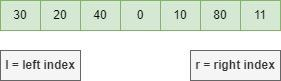• Now we need to divide the array containing 7 elements into two arrays of size having 4 elements on left and 3 elements on right.

• After dividing the array into two halves. It will look like below.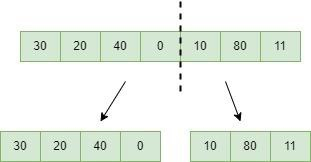• Divide the array into another halves, until the array no longer be divided.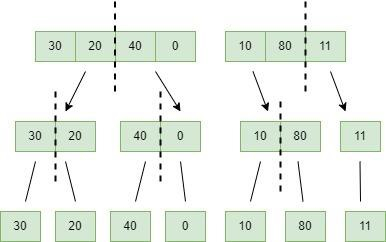• After dividing all the elements into smallest units, now compare the elements based on size of elements.

• Initially compare the element of the array in each list, then sort and merge them into another list.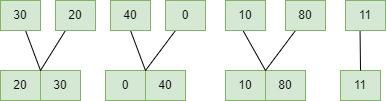• After performing all the merging’s, this will be the following list.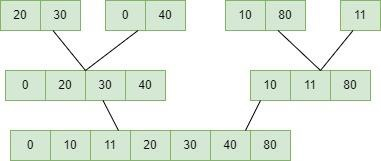### Algorithm

Let’s look into the algorithm −

• Declare an array, left index, right index and middle variable.

• Perform the merge sorting.

mergesort(array, left index, right index)

if left index > right index

return

mid= (left index + right index) / 2

mergesort(array, left index, mid) // First half

mergesort(array, mid+1, right index) // Second half

merge(array, left index, mid, right index) // merge two halfes sorted in above steps

### Example

In the below example, to perform the merge sort we have created an array having elements in a random order. And by using Merge Sort algorithm we have sorted the array elements in ascending order.

<!DOCTYPE html>
<html>
<title>Merge Sort</title>
<script>
function merge_Arrays(left_sub_array, right_sub_array) {
let array = []
while (left_sub_array.length && right_sub_array.length) {
if (left_sub_array < right_sub_array) {
array.push(left_sub_array.shift())
} else {
array.push(right_sub_array.shift())
}
}
return [ ...array, ...left_sub_array, ...right_sub_array ]
}
function merge_sort(unsorted_Array) {
const middle_index = unsorted_Array.length / 2
if(unsorted_Array.length < 2) {
return unsorted_Array
}
const left_sub_array = unsorted_Array.splice(0, middle_index)
return merge_Arrays(merge_sort(left_sub_array),merge_sort(unsorted_Array))
}
unsorted_Array = [39, 28, 44, 4, 10, 83, 11];
document.write("The sorted array will be: ",merge_sort(unsorted_Array));
</script>
<body>
</body>
</html>


### Note

• The merge sort algorithm performs slower when compared to the other sorting algorithms.

• It iterates through the entire process of sorting even though the array is already sorted.

• This algorithm also requires extra memory space of 0(n) for temporary array.